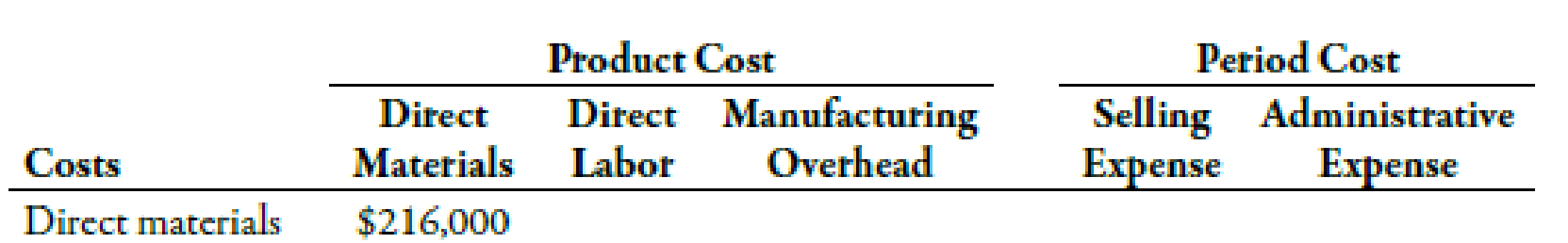Chapter 2, Problem 39E### Managerial Accounting: The Corners...

7th Edition
Maryanne M. Mowen + 2 others
ISBN: 9781337115773

#### Solutions

Chapter
Section### Managerial Accounting: The Corners...

7th Edition
Maryanne M. Mowen + 2 others
ISBN: 9781337115773
Textbook Problem
16 views

# Cost ClassificationLoring Company incurred the following costs last year:Required: 1. Classify each of the costs using the following table format. Be sure to total the amounts in each column. Example: Direct materials, $216,000.2. What was the total product cost for last year? 3. What was the total period cost for last year? 4. If 30,000 units were produced last year, what was the unit product cost? 1. To determine Classify each cost as a product cost or period cost. Explanation Product Cost: The amount of cost whether direct or indirect incurred by the company in order to produce a product is known as product cost. It also means the cost incurred by the company in acquiring a product and making it available for sale. Period costs can be defined as the costs, which are not associated with the process of manufacturing. Selling and distribution expenses are examples of period costs. The classification of the costs is given below:  Product cost Period cost Costs Direct materials ($) Direct labor ($) Manufacturing Overhead ($) Selling expense ($) Adminis-trative expense ($) Direct materials 216,000 Factory rent 24,000 Direct labor 120,000 Factory utilities 6,300 Supervisi...

2.

To determine

Calculate the amount of product cost for the previous year.

3.

To determine

Calculate the amount of period cost for the last year.

4.

To determine

Calculate the amount of unit product cost.

### Still sussing out bartleby?

Check out a sample textbook solution.

See a sample solution

#### The Solution to Your Study Problems

Bartleby provides explanations to thousands of textbook problems written by our experts, many with advanced degrees!

Get Started